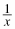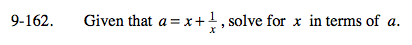### Home > PC > Chapter 9 > Lesson 9.3.5 > Problem9-162

9-162.

Given that a = x +, solve for x in terms of a. Homework Help ✎Multiply each term by x.

Move all terms to one side and set equal to 0.

$x=\frac{-(-a)\pm \sqrt{(-a)^2-4(1)(1)}}{2(1)}$